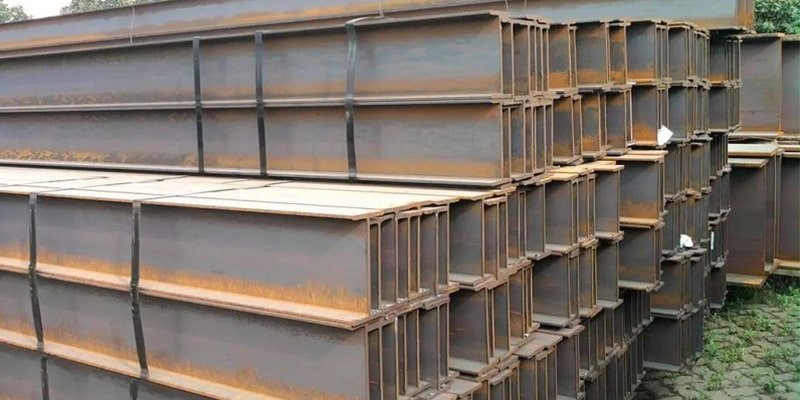# H Beam & I Beam Weight Calculator & Chart (Free to Use)

## What Is I Beam

I-beam is also called steel beam (Universal Beam), which is a long strip of steel with a cross-section of I-shaped. I-beam is divided into ordinary I-beam and light I-beam.

## What Is H BeamH-beam is developed from the optimization of I-shaped steel. The name is derived from the fact that its section is the same as the English letter H. It is an economic section high-efficiency profile with more reasonable strength-to-weight ratio and more optimized cross-sectional area distribution.

Each part of the H-shaped steel is arranged at a right angle, so it has the advantages of strong bending resistance, cost saving, simple construction and lightweight in all directions.

It is often used in large buildings that require large interception capacity and good cross-section stability, such as high-rise buildings and workshops. In addition, it is also widely used in ships, bridges, lifting and transportation machinery, brackets, equipment foundations, foundation piles etc.

## H Beam vs. I Beam

As for the difference between H-beam and I-beam, you can refer to the article below.

## H Beam Weight & I Beam Weight Calculation

In this article, we mainly discuss how to calculate the weight of H-beam and I-beam.

For the convenience of calculation, we have created two calculators: H-beam weight calculator and I-beam weight calculator.

By using these two calculators, you can easily calculate the weight of H-beam and I-beam.

Of course, for more calculations of different metal weights, you can refer to the following article.

In addition, we also made a press brake tonnage calculator and press force calculator. If you are interested, you can use it by clicking the link.

Now, start using the calculator to calculate the profile weight.If you are tired of using a calculator to calculate the weight of H beam and I beam, you can refer to the following H-beam weight table and I-beam weight table. It allows you to check the H-beam and I-beam weights of different sizes more quickly.